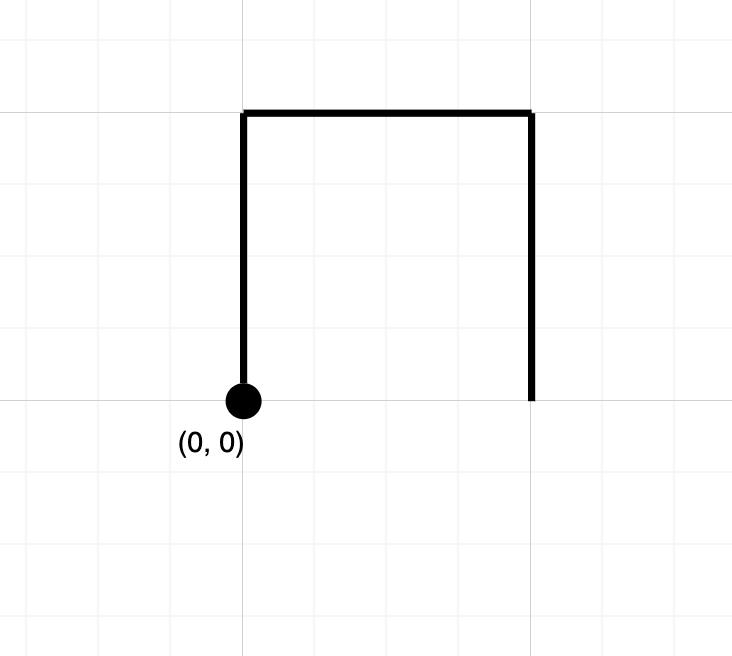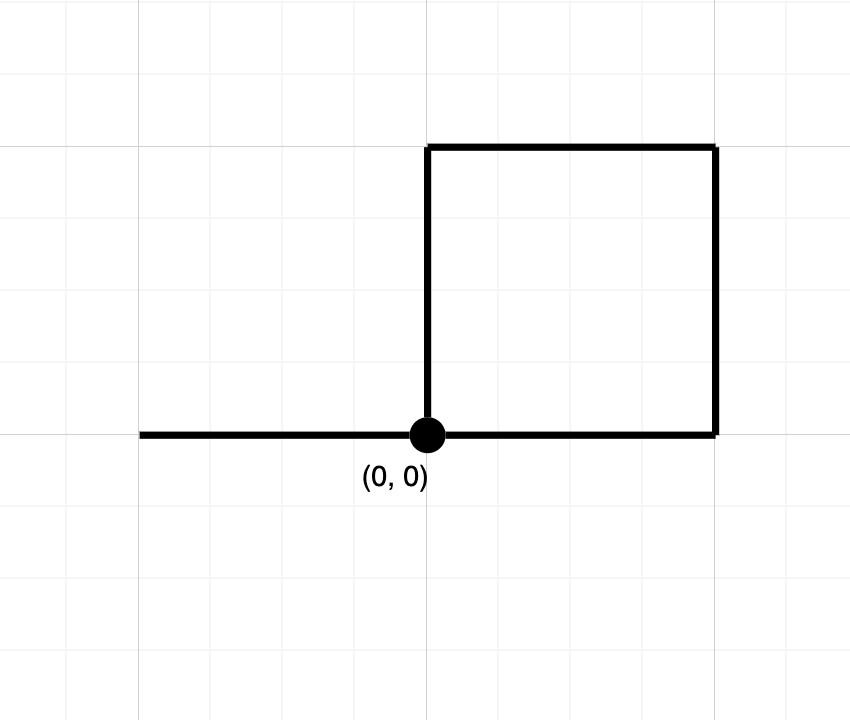LeetCode - Strings - Path Crossing

Given a string path, where path[i] = 'N''S''E' or 'W', each representing moving one unit north, south, east, or west, respectively. You start at the origin (0, 0) on a 2D plane and walk on the path specified by path.

Return True if the path crosses itself at any point, that is, if at any time you are on a location you've previously visited. Return False otherwise.

Example 1:Input: path = "NES"
Output: false
Explanation: Notice that the path doesn't cross any point more than once.

Example 2:Input: path = "NESWW"
Output: true
Explanation: Notice that the path visits the origin twice.

Constraints:

• 1 <= path.length <= 10^4
• path will only consist of characters in {'N', 'S', 'E', 'W}

class Solution {
public boolean isPathCrossing(String path) {
int x = 0;
int y = 0;
List<String> pathList = new ArrayList<String>();
for(int i=0;i<path.length();i++){
//System.out.println(path.charAt(i));
String direction = path.charAt(i)+"";
if("N".equals(direction)){
y++;
}else if("S".equals(direction)){
y--;
}else if("E".equals(direction)){
x++;
}else{
x--;
}
if(pathList.contains(x+""+y))
return true;
else

}
//System.out.println(pathList);
return false;
}
}